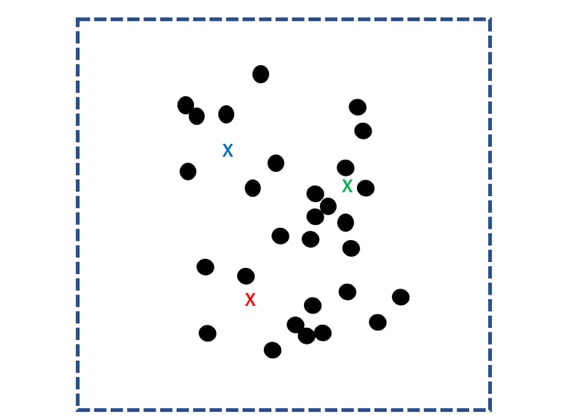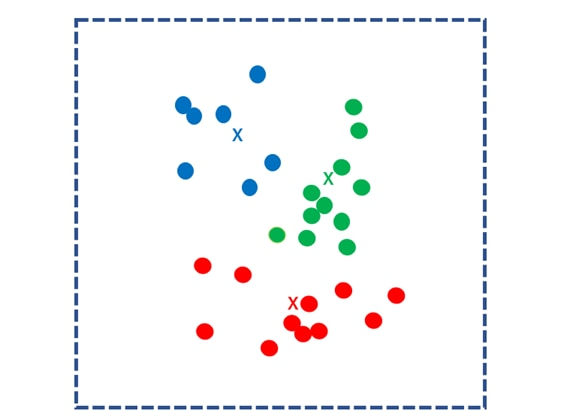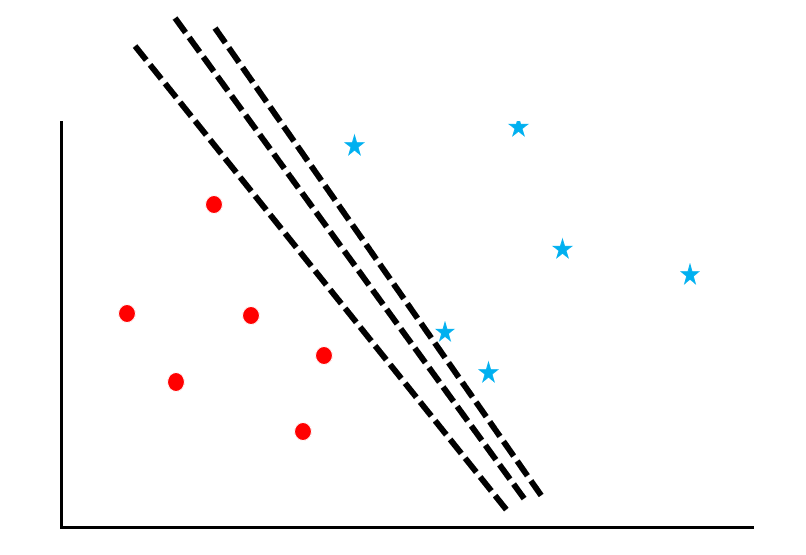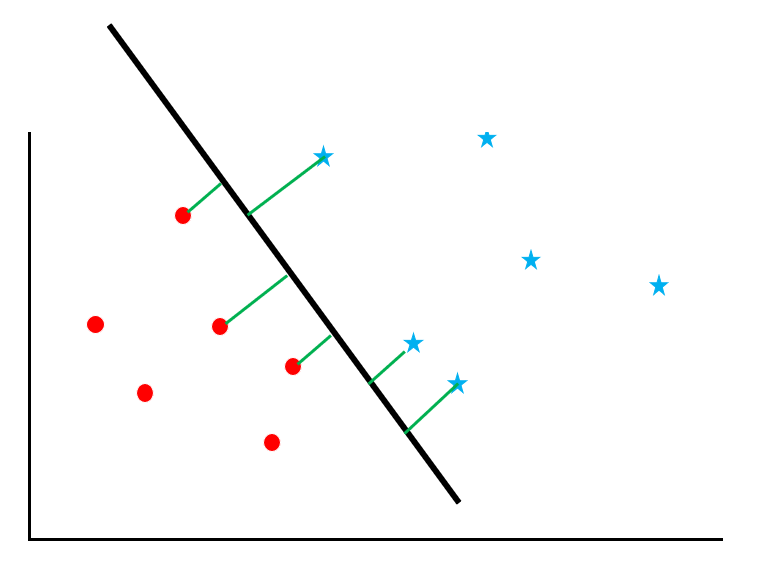Tuesday, October 3, 2023
HomeAIMachine Learning12 representative machine learning algorithms | Introducing machine learning learning methods!

# 12 representative machine learning algorithms | Introducing machine learning learning methods!

• 12 representative algorithms of machine learning
• (1) Linear regression
• (2) Logistic regression
• (3) Decision tree
• ④ Random forest
• (5) K nearest neighbor method (KNN)
• ⑥K-means
• ⑦ Support Vector Machine (SVM)
• ⑧ Support Vector Regression (SVR)
• ⑨ Naive Bayes
• (10) CNN
• ⑪RNN
• ⑫GANs
• 4(+1) Learning Techniques in Machine Learning
• (1) Supervised learning
• (2) Unsupervised learning
• ③ Reinforcement learning
• ④Semi-supervised learning
• What is the relationship between deep learning and machine learning algorithms?
• How can I try machine learning algorithms myself?
• summary

## 12 representative algorithms of machine learning

From here, we will introduce the representative algorithms of machine learning. The algorithm introduced here is as follows.

1. linear regression
2. logistic regression
3. decision tree
4. random forest
5. K nearest neighbors (KNN)
6. K-means
7. Support Vector Machine (SVM)
8. Support Vector Regression (SVR)
9. Naive Bayes
10. CNN
11. RNN
12. GANs

I will explain each.

### (1) Linear regression

Regression analysis is a method of predicting the “objective variable” you want to predict based on other “explanatory variables”.

When there is only one explanatory variable, it is called simple regression analysis. When the objective variable y is the dependent variable and the explanatory variable x is the independent variable, simple regression analysis can be expressed as a linear function of the form “y=ax+b” with a and b as parameters.

When there are multiple explanatory variables, it is called multiple regression analysis.

There are two types of regression analysis: linear regression and nonlinear regression.

This is an intuitive explanation that lacks rigor, but a regression analysis that can linearly express the relationship shown in the figure above, in other words, the relationship between data is called “linear regression.”

### (2) Logistic regression

Logistic regression is an analytical technique developed in the field of medicine.

It is an application of a technique called linear regression, and although it has the word regression in its name, it is actually a technique used for classification problems.

Basically, this means dealing with problems where the objective variable can be classified as either “Yes (= 1)” or “No (= 0)”.

This method infers qualitative variables (things that are difficult to express numerically, such as “likes and dislikes”) from quantitative variables (things that can be expressed numerically). Predict the probability that is “1”.

Therefore, even if it is difficult to understand with multiple regression analysis, such as a numerical value of 1 or more, or a negative value, it is easy to understand when looking at the analysis results because the probability can always be found in the range of 0 to 1 in logistic regression analysis. It is characterized by

### (3) Decision tree

There are two types of decision trees, classification trees and regression trees, and these are algorithms that learn by setting a threshold.

It looks like the tree-shaped graph below. It is used when making decisions, including risk management.

Created by AINOW editorial department

### ④ Random forest

Random forest uses the results of multiple decision trees to output the final result by taking the majority vote and averaging.

By using this method, even if there is a decision tree with poor accuracy, the results can be supplemented with other decision trees, so the overall accuracy can be improved.

Created by AINOW editorial department

### (5) K nearest neighbor method (KNN)

Algorithm for a typical classification problem. It determines to which class unknown data belongs to a group of classified data scattered on coordinates.

Extract k pieces of data from the unknown data in descending order of distance, and distribute the unknown data to the class with the largest number among the k pieces of data.

Determine to which class the unknown data belongs to the already labeled data group. In this example, there are three classes: the red circle class, the blue star class, and the green diamond class.
With k=3, extract 3 data in descending order of distance from unknown data. In this example, we have 1 blue star and 2 green diamonds, so we decide by majority vote that this unknown data belongs to the green diamond class.

### ⑥K-means

The k-means method is the most commonly used clustering algorithm.

First, randomly determine k centroid points for the scattered data group and use them as the core.Then, the distances to the k nuclei are calculated for all data and grouped into the closest nuclei. This group is called a “cluster”.Next, find the center of gravity for each cluster and use it as the new k kernels. Repeat the same process to separate each data into the nearest centroid clusters.Repeat this process until the center of mass no longer moves. The calculation ends when the centroid point is no longer updated.

### ⑦ Support Vector Machine (SVM)

A support vector machine is an algorithm that calculates “margin maximization” for a data group. Let’s follow the process with reference to the diagram.

Let’s consider the problem of separating red circles and blue stars from scattered data with a “boundary line”. However, as you can see in this figure, there are many ways to draw the line.Now consider “maximizing the support vector margin”.

Support vectors refer to the data near the border, and margin refers to the distance between the border and the data. The green line in the figure is the margin.### ⑧ Support Vector Regression (SVR)

Support Vector Regression is a regression version of Support Vector Machine, a technique that can solve nonlinear problems with many variables.

Just as SVM learns by maximizing margins and reducing misestimated samples, SVR learns by minimizing weights and minimizing errors.

### ⑨ Naive Bayes

This method uses Bayes’ theorem, a famous theorem used as a basis in statistics, to calculate the probability of being classified into each class and output the class with the highest probability as a result, solving the classification problem. It is a model for

### (10) CNN

CNNs are very effective models for image-related tasks.

The model used for AI that recognizes cats developed by Google in 2012 is also CNN.

A major feature of CNN is the convolutional and pooling layers.

I won’t go into how these layers operate (for those who are interested, please read O’Reilly’s ” Deep Learning from Scratch “). By layering, it becomes possible to extract more abstract information from the input image.

Based on the information extracted in this way, high performance is achieved even for complex tasks.

### ⑪RNN

RNNs are effective models for tasks such as time-series data analysis and natural language processing.

Time-series data refers to data whose values ​​change over time, such as stock prices.

Natural language processing, on the other hand, aims at understanding the meaning of language with human ambiguity and translating from one language to another.

For time-series data, just as the relationship between information at a certain time and information at an earlier time is important, so for natural language, the relationship between a word in a sentence and the word before it (for example, after the subject is verb comes) becomes important.

Since RNN can perform calculations that consider such past information, it is effective for tasks such as those described above.

### ⑫GANs

The last one to introduce is GAN. Generative adversarial networks are currently a successful method, mainly in the field of imaging.

A GAN consists of two networks, one called a generator and the other called a discriminator.

The generator repeats output and learning so that the discriminator makes mistakes, and the discriminator learns so that it can properly detect fakes.

By playing cat-and-mouse, these two will raise each other’s accuracy more and more.

GANs, in which the model automatically improves accuracy, have produced surprising results that have been difficult to achieve with other methods.

## 4(+1) Learning Techniques in Machine Learning

### (1) Supervised learning

Supervised learning is a method of learning with correct labels given to the training data.

Supervised learning uses teacher data as known information for learning, and constructs regression models and classification models that can deal with unknown information.

A computer is trained on a large number of photos pre-labeled as “dog” or “cat” (teaching data) to build a model.

Given an unlabeled photo, the model will output the label attached to the trained image with the closest features to that image. In other words, this model will be able to detect whether a given image is a “dog” or a “cat”

### (2) Unsupervised learning

Unsupervised learning is a method of learning without assigning correct labels to the training data.

It is mainly used for data grouping ( clustering ).

Even if there are no labels in the training data, training a computer with a large number of images enables grouping and summarization of information based on image features (eg size, color, shape).

### ③ Reinforcement learning

Reinforcement learning does not learn from given data, but rather takes action on its own and, through trial and error, learns actions and choices that provide rewards (evaluations).

An example of reinforcement learning is dog training. If the dog touches you, give him a treat. If the dog repeats the “Hand” trial, the dog will learn that “handing ⇒ getting food”.

Taking the walking of a robot as an example, if the “distance walked” is the reward, “extending the walking distance by trial and error in how to move the hands and feet” corresponds to reinforcement learning.

### ④Semi-supervised learning

In semi-supervised learning, the annotation is partially automated.

For example, let’s say you want to use semi-supervised learning to determine the gender of a human image. First, humans annotate.

Next, from the annotated data, it learns that “If it has this feature, it will be classified as male, and if it is this, it will be classified as female.”

It then predicts the labels of the rest of the image data and adds the high confidence ones to the data (bootstrap method).

If this works well, it will be possible to reduce the cost of creating training data.

## What is the relationship between deep learning and machine learning algorithms?

Deep learning, also known as deep learning, differs from conventional machine learning in that the machine learns features. Both supervised and unsupervised learning exist.

For example, suppose you create an AI that can distinguish between dogs and cats. Traditionally, humans have specified where to focus. For example, the shape of the ears or the presence of a beard.

Deep learning, on the other hand, allows machines to automatically learn what is important. As a result, it may be more accurate than using human-specified features.

## How can I try machine learning algorithms myself?

Colab (formally called “Colaboratory”) is a service that allows you to write and run Python on your browser . Colab has the following advantages:

• No need for environment construction
• Share easily

Colab streamlines the work of everyone, from students to data scientists to AI researchers.

## summary

In this article, we will discuss machine learning algorithms. I also explained the differences between algorithms, but the best way to deepen your understanding of machine learning is to actually try it yourself.

With Google Colab, anyone can run machine learning code written in Python from a PC browser, so please try it while referring to the demonstration article.

RELATED ARTICLES

Recommended